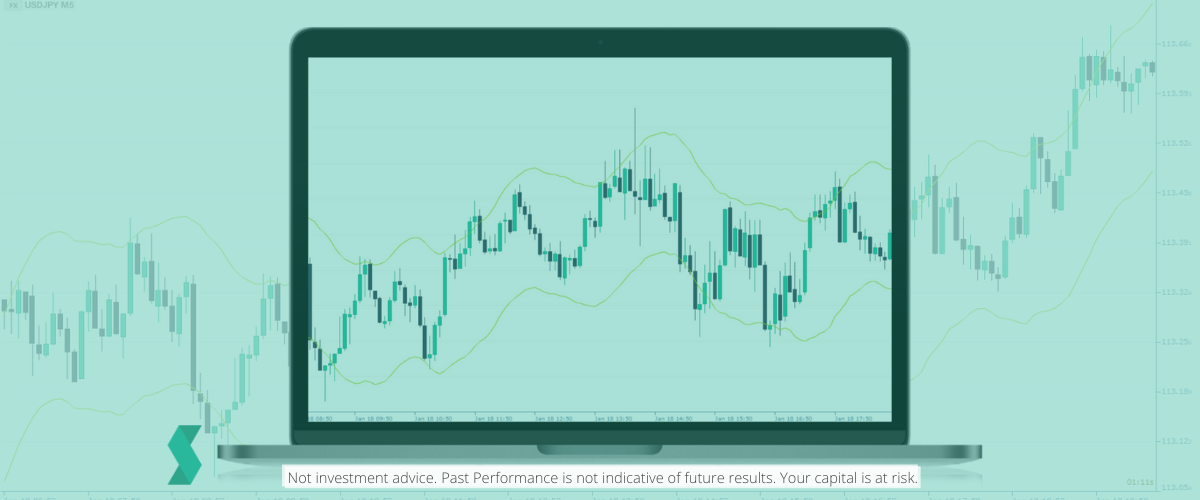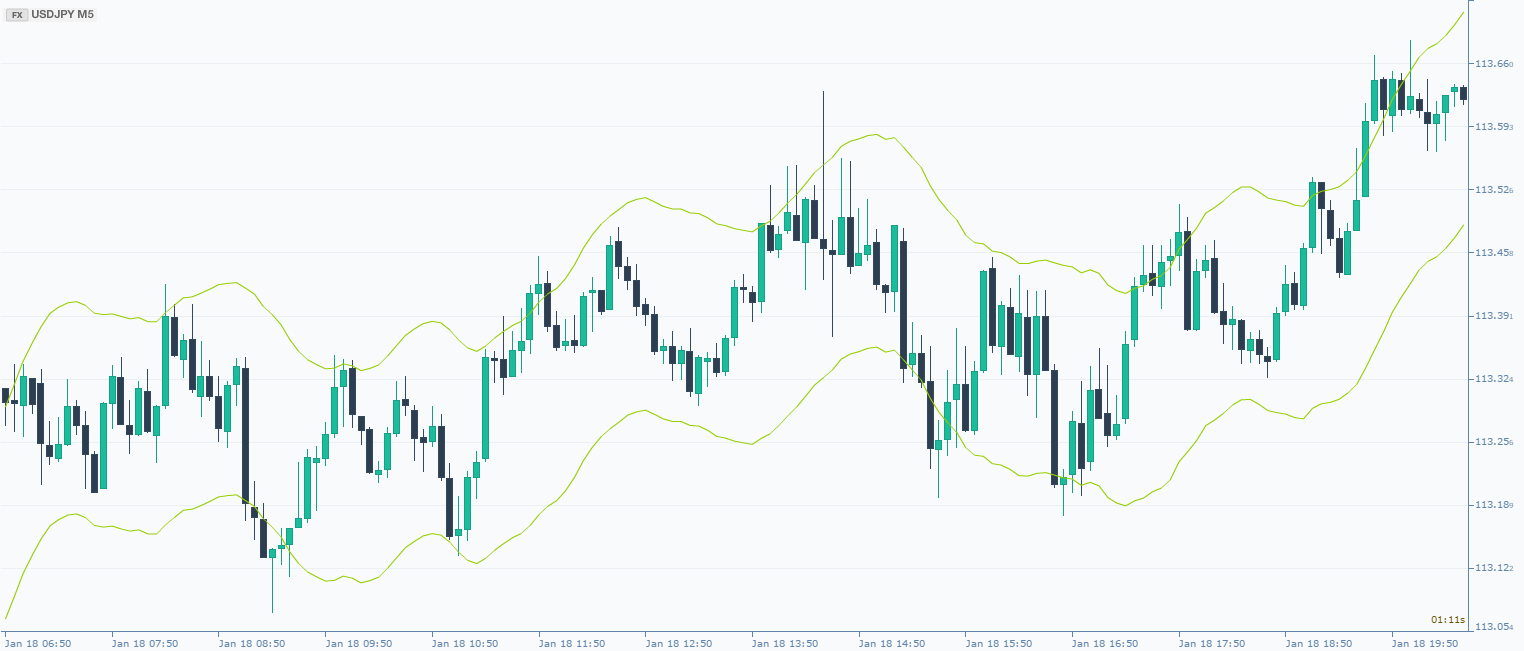# Moving average envelopesMoving Average Envelopes is a percentage-based indicator which places bands above and below a moving average (MA). The MA can be a based on the closing, opening, highs or lows using either simple or moving averages. Each envelope can then be set at the same percentage, above or below the moving average, with these visual lines creating parallel bands that then follows the price action. If you like using moving averages, then perhaps this could be a more advanced technique for you to consider. In addition, Moving Average Envelopes are not merely a trend following indicator as the envelopes can also highlight overbought and oversold levels - providing potential entry or exit signals.

### The indicator has two components:

Moving average
We can adjust the moving average so that it can be based on the closing or opening prices or even the highs or the lows (and either simple or exponential)
Upper and Lower bands
The moving average minus / plus, depending on the side, of the user-defined percentage.The calculation of the moving average is simple; it weights each data point (prices) and selects the number of time periods (which by default is 14) for the moving average. To create the envelope indicator, the calculation will be supplemented by a percentage that will be shown as two shifted lines on the chart.

Since the prices of instruments tend to stay within the range represented by an envelope, the theory is that they will then continue to bounce between the upper and lower thresholds, which would give the trader good buying and selling signals (if you know the market is overbought and likely to come down you would naturally sell, and vice versa).

Skilling Summary:
If you like using moving averages, then you should definitely explore Moving Average Envelopes. They provide a slightly more advanced method for providing trading signals than using just traditional moving averages - so if you are a trend following trader, then they might be very helpful.

## Related Articles

### Explanation of different types of candlestick patterns

If you want to try predicting the markets using a candlestick chart, here are some of the most popular and useful patter...

### What is Ichimoku Cloud and how to read it?

The Ichimoku Cloud consists of five lines plotted on a candlestick chart. Being originally a Japanese indicator, many of...

### RSI: Relative Strength Index - Definition

The Relative Strength Index (RSI) is an extremely popular momentum indicator, perhaps the most well-known one by J. Well...